# Square and Square Roots Class 8 notes

In this page we will explain the topics for the chapter 6 of Square and Square Roots Class 8 Maths.We have given quality Square and Square Roots Class 8 notes along with video to explain various things so that students can benefits from it and learn maths in a fun and easy manner, Hope you like them and do not forget to like , social share and comment at the end of the page.

Table of Content

## Square Number

if a natural number m can be expressed as n2, where n is also a natural
number, then m is a square number
Example
1=12
4=22
The numbers 1, 4, 9, 16 ... are square numbers. These numbers are also called perfect squares.

## Important point to Note for Square Numbers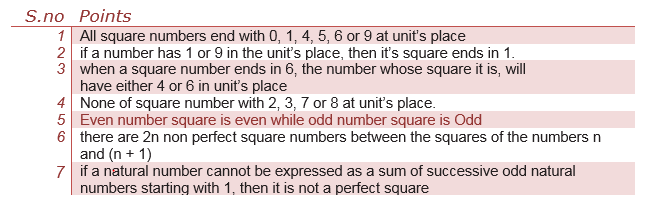## How to find the square of Number easily

### Identity method

We know that
(a+b)2 =a2 +2ab+b2
Example
232= (20+3)2 =400+9+120=529

### Special Case

(a5)2
= a(a + 1) hundred + 25
Example
252=2(3) hundred +25=625

Watch this tutorial for an example of square numbers

## Pythagorean triplets

For any natural number m > 1, we have (2m)2 + (m2 – 1)2 = (m2 + 1)2
So, 2m, m2 – 1 and m2 + 1 forms a Pythagorean triplet
Example
6,8,10
62 +82 =102
Lets take some solved example on it
Solved Example
Find a Pythagorean triplet in which one member is 12.
Solution:
If we take m2 – 1 = 12
Then, m2 = 12 + 1 = 13
Then the value of m will not be an integer.
So, we try to take m2 + 1 = 12. Again m2 = 11 will not give an integer value for m.
So, let us take 2m = 12
then m = 6
Thus, m2 – 1 = 36 – 1 = 35 and m2 + 1 = 36 + 1 = 37
Therefore, the required triplet is 12, 35, 37

## Square Root

Square root of a number is the number whose square is given number
So we know that
m=n2
Square root of m
√m =n
Square root is denoted by expression √

## How to Find Square root

### Finding square root through repeated subtraction

We know sum of the first n odd natural numbers is n2. So in this method we subtract the odd number starting from 1 until we get the reminder as zero. The count of odd number will be the square root
Consider 36
Then,
(i) 36 – 1 = 35 (ii) 35 – 3 = 32 (iii) 32 – 5 = 27 (iv) 27 – 7 = 20
(v) 20 – 9 = 11 (vi)11 – 11 = 0
So 6 odd number, Square root is 6

## Finding square root through prime factorization

This method, we find the prime factorization of the number.
We will get same prime number occurring in pair for perfect square number. Square root will be given by multiplication of prime factor occurring in pair
Consider
81
81= (3×3)×(3×3)
√81= 3×3=9
Example
Find the square root of 57600.
Solution: Write 57600 = 2 × 2 × 2 × 2 × 2 × 2 × 2 × 2 × 5 × 5×3×3
Therefore 57600= 2 × 2 × 2 × 2 × 5×3 = 240

## Finding square root by division method

This can be well explained with the example
Step 1 Place a bar over every pair of digits starting from the digit at one’s place. If the number of digits in it is odd, then the left-most single digit too will have a bar. So in the below example 6 and 25 will have separate bar
Step 2 Find the largest number whose square is less than or equal to the number under the extreme left bar. Take this number as the divisor and the quotient with the number under the extreme left bar as the dividend. Divide and get the remainder
In the below example 4 < 6, So taking 2 as divisor and quotient and dividing, we get 2 as reminder
Step 3 Bring down the number under the next bar to the right of the remainder.
In the below example we bring 25 down with the reminder, so the number is 225
Step 4 Double the quotient and enter it with a blank on its right.
In the below example, it will be 4
Step 5 Guess a largest possible digit to fill the blank which will also become the new digit in the quotient, such that when the new divisor is multiplied to the new quotient the product is less than or equal to the dividend.
In this case 45 × 5 = 225 so we choose the new digit as 5. Get the remainder.
Step 6 Since the remainder is 0 and no digits are left in the given number, therefore the number on the top is square root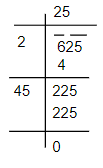In case of Decimal Number, we count the bar on the integer part in the same manner as we did above, but for the decimal part, we start pairing the digit from first decimal part.
Example Find the square root of 870.25
Solution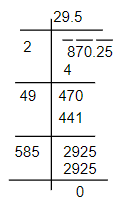Example
Find the Square root of 841
Solution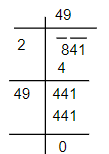## Estimating Digits in the Square Root

if a perfect square is of n-digits, then its square root will have n/2 digits if n is even or (n+ 1)/2
if n is odd
Or
We can create the pair in the number, the Number of pair gives the number of digit in the square root
Example
Find the number of digits in the square root of the following numbers.
(i) 25921
(ii) 37249
Solution
Here n is odd, so (n+1)/2 = 3
So three digits will be present in square root

## Frequently asked Questions on CBSE Class 8 Maths Chapter 6: Square and Square Roots

### What do you mean by perfect square??

Perfect square is a number which can be expressed as square of any integers $2 \times 2 =4$ $3 \times 3 =9$

### Is 33 a perfect square?

33 is not perfect square as it can be expressed as square of an integers

### Is 256 a perfect square?

256 is a perfect square as $256=16^2$

### Is 1000 a perfect square?

Cube root of a number can be found using prime factorization method

1000 is not perfect square as it can be expressed as square of an integers

### How to estimate the unit digit of square root?

You can the below chart to estimate the unit digit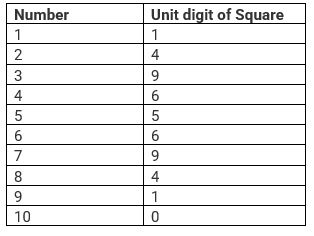### What is the easiest way to find the square root of a number?

You can below trick to find the square roor quickly Step 1: if a perfect square is of n-digits, then its square root will have n/2 digits if n is even or (n+ 1)/2 if n is odd. So for three digit number, square root will be 2 digit, 4 digit number square root will be 2 digit number,5 digit number square root will be 3 digit number Step 2 We can predict the Unit digit of the square root from the unit digit of the number. If the unit digit is 4, then the square root unit digit can be 2 or 8 Step 3: For Three Digit Number (i)Now we take the right most number and let call it n (ii) Now we determine two squares this number lies, $a^2$ < n < $b^2$. We choose the smallest one i.e a. (iii) If we have two option for unit digit,then we can decide using the below method (iv) We multiply a and b ,if ab > n, then take the lessor number else take the bigger number Example 784 Here Unit of square root can be 2 or 8 Now $2^2 < 7> 3^2$, so we choose the smaller number Now the square root can be 22 or 28 Now $2 \times 3 =6$ < 7, so we need to take bigger number $\sqrt {784} =28$ Step 4: For four Digit Number (i)Now we take the right most number 2 digit and let call it n (ii) Now we determine two squares this number lies, $a^2$ < n < $b^2$. We choose the smallest one i.e a. (iii) If we have two option for unit digit,then we can decide using the below method (iv) We multiply a and b ,if ab > n, then take the lessor number else take the bigger number Example 6889 Here Unit of square root can be 3 or 7 Now $8^2 < 68 > 9^2$, so we choose the smaller number 8 Now the square root can be 83 or 87 Now $8 \times 9 =72$ > 68, so we need to take less number $\sqrt {6889} =83$ Step 4: For five Digit Number (i)Now we take the right most number 3 digit and let call it n (ii) Now we determine two squares this number lies, $a^2$ < n < $b^2$. We choose the smallest one i.e a. (iii) If we have two option for unit digit,then we can decide using the below method (iv) We multiply a and b ,if ab > n, then take the lessor number else take the bigger number Example 10816 Here Unit of square root can be 4 or 6 Now $10^2 < 108 > 11^2$, so we choose the smaller number 10 Now the square root can be 104 or 106 Now $10 \times 11 =111$ > 108, so we need to take less number $\sqrt {10816} =104$

100489

## Summary

Here is Square and Square Roots Class 8 notes Summary
1. If a natural number m can be expressed as $n^2$ , where n is also a natural number i.e $m =n^2$ then m is a square number.
2. All square numbers end with 0, 1, 4, 5, 6 or 9 at unit’s place.
3. Square numbers can only have even number of zeros at the end.
4. Square root is the inverse operation of square. There are two integral square roots of a perfect square number.Positive root is denoted by $\sqrt$